Courses

# Previous Year Questions (2014-19) - Chemical Kinetics Notes | EduRev

## NEET : Previous Year Questions (2014-19) - Chemical Kinetics Notes | EduRev

The document Previous Year Questions (2014-19) - Chemical Kinetics Notes | EduRev is a part of the NEET Course Chemistry 28 Years Past year papers for NEET/AIPMT Class 12.
All you need of NEET at this link: NEET

Q.1. If the rate constant for a first order reaction is k, the time (t) required for the completion of 99% of the reaction is given by:    (2019)
A: t = 0.693/k
B: t = 6.909/k
C: t = 4.606/k
D: t = 2.303/k
Ans:
C
First order rate constant is given as,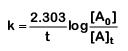99% completed reaction,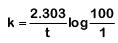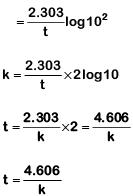Q.2. For the chemical reaction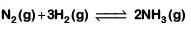The correct option is:    (2019)
A: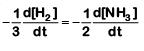B: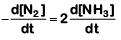C: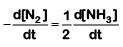D:Ans:
C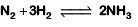Rate of reaction is given as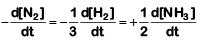Q.3. When initial concentration of the reactant is doubled,the half-life period of a zero order reaction    (2018)
A: is halved
B: is doubled
C: is tripled
D: remains unchanged
Ans:
B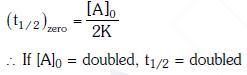Q.4. The correct difference between first- and second-order reaction is that    (2018)
A: the rate of a first-order reaction does not depend on reactant concentration; the rate of a second order reaction does depend on reactant concentrations.
B: the half-life of a first-order reaction does not depend on [A]0; the half-life of a second-order reaction does depend on [A]0
C: a first-order reaction can be catalyzed;a second-order reaction cannot be catalyzed.
D: the rate of a first-order reaction does depend on reactant concentrations; the rate of a second-order reaction does not depend on reactant concentrations
Ans:
B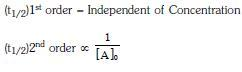Q.5. Mechanism of a hypothetical reaction    (2017)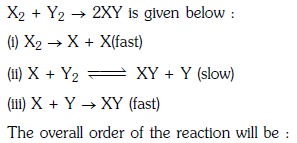A: 2
B: 0
C: 1.5
D: 1
Ans: C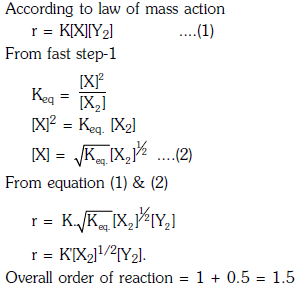Q.6. A first order reaction has a specific reaction rate of 10–2 sec–1. How much time will it take for 20g of the reactant to reduce to 5 g ?    (2017)
A: 138.6 sec
B: 346.5 sec
C: 693.0 sec

D: 238.6 sec
Ans:
A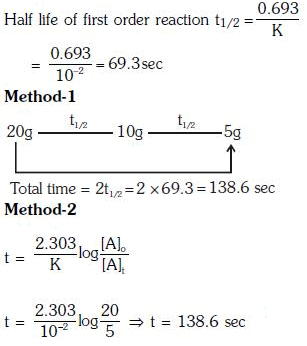Q.7. The rate of a first-order reaction is 0.04 mol ℓ-1 s-1 at 10 seconds and 0.03 mol ℓ-1 s-1 at 20 seconds after initiation of the reaction. The half-life period of the reaction is :    (2016)
A: 54.1 s
B: 24.1 s
C: 34.1 s
D: 44.1 s
Ans:
B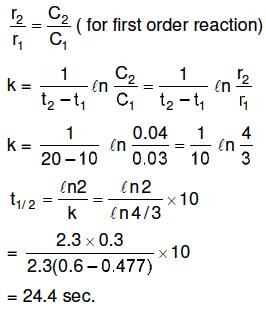Q.8. The activation energy of a reaction can be determined from the slope of which of the following graphs ?    (2015)
A: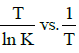B: ln K vs. T
C: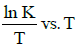D: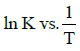Ans:
D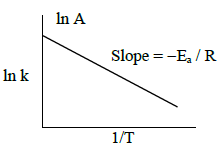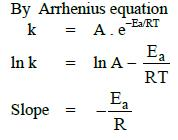Q.9. When initial concentration of a reactant is doubled in a reaction, its half-life period is not affected. The order of the reaction is :    (2015)
A: More than zero but less than first
B: Zero
C: First
D: Second
Ans:
C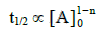For first order reaction, half-life period is not depends upon initial concentration.

Offer running on EduRev: Apply code STAYHOME200 to get INR 200 off on our premium plan EduRev Infinity!

,

,

,

,

,

,

,

,

,

,

,

,

,

,

,

,

,

,

,

,

,

;